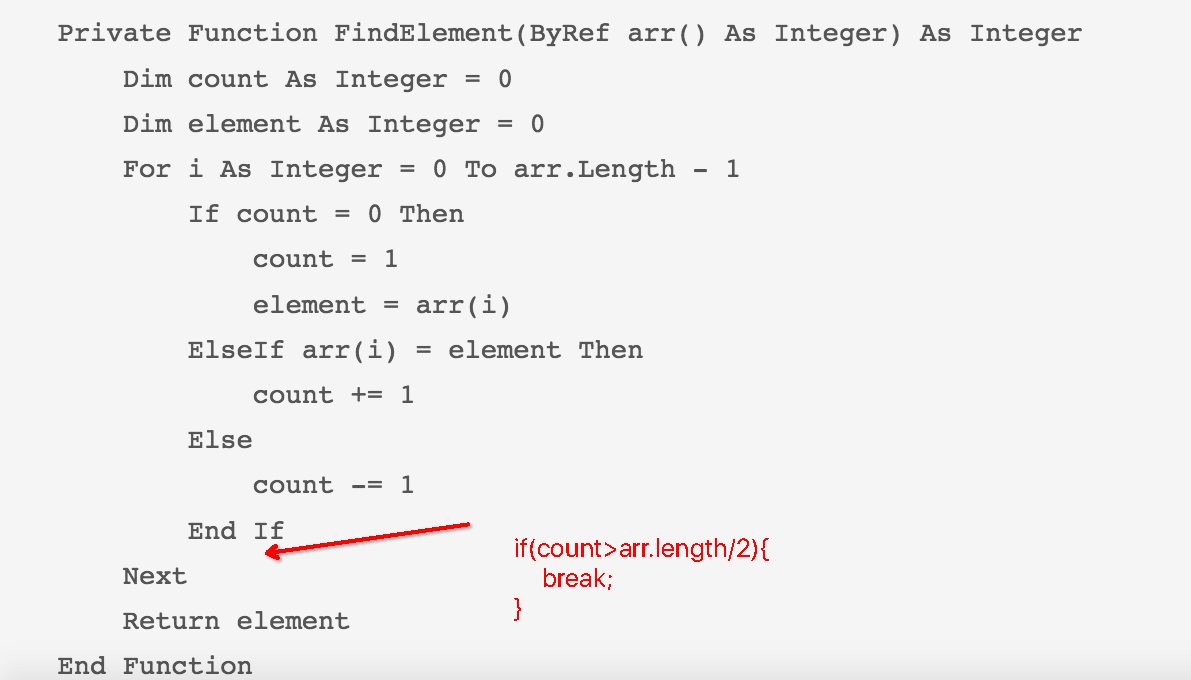• ## 众数

2017-12-04 10:05:24
众数
 众数
Problem Description

众数是指在一组数据中，出现次数最多的数。例如：1, 1, 3 中出现次数最多的数为 1，则众数为 1。  给定一组数，你能求出众数吗？

Input  输入数据有多组（数据组数不超过 50），到 EOF 结束。  对于每组数据：  第 1 行输入一个整数 n (1 <= n <= 10000)，表示数的个数。  第 2 行输入 n 个用空格隔开的整数 Ai (0 <= Ai <= 1000)，依次表示每一个数。

Output  对于每组数据，在一行中输出一个整数，表示这组数据的众数。  数据保证有唯一的众数。  Example Input

3
1 1 3
5
0 2 3 1 2

Example Output

1
2


代码：
#include <stdio.h>
#include <stdlib.h>

int main()
{
int n;
while(scanf("%d", &n) != EOF)
{
int i, a = {0}, count = {0}, max, index;
for(i = 0; i < n; i++)
{
scanf("%d", &a[i]);
count[a[i]]++;
}
max = count[a];
index = a;
for(i = 0; i < n; i++)
{
if(count[a[i]] > max)
{
max = count[a[i]];
index = a[i];
}
}
printf("%d\n", index);
}
return 0;
}


展开全文• 最终剩下的仍然是众数，更何况每次进行相邻数据相抵的时候还存在两个不同非众数的相抵。所以只需要O（N）就把众数找到了。 2、这里面很巧妙的地方在哪里？ 众数占绝对优势。 这里面有个思考非常常用，就是...
今天的算法题目是：
Given an array of size n, find the majority element. The majority element is the element that appears more than ⌊ n/2 ⌋ times.
You may assume that the array is non-empty and the majority element always exist in the array.
Example 1:
Input: [3,2,3] Output: 3 Example 2:
Input: [2,2,1,1,1,2,2] Output: 2
那么如何设计出高效的算法呢？你如果没有想到时间复杂度为N的算法，那么别费劲了直接参考一下答案吧。
答案：逆向思考：
1、答案的思路是什么？
这里众数的个数大于数组的一半，在这种众数占有绝对优势的情况下，其实全局来看其实问题就设计到了两个数：是众数、不是众数。所以呢，我们就拿众数跟非众数一个一个得相抵消。最终剩下的仍然是众数，更何况每次进行相邻数据相抵的时候还存在两个不同非众数的相抵。所以只需要O（N）就把众数找到了。
2、这里面很巧妙的地方在哪里？
众数占绝对优势。
这里面有个思考非常常用，就是用相抵的办法去等同地降低问题复杂度。这个思路在以后还会用到。
3、为什么你没想到答案？
正向思维太强，缺乏一定的逆向思维。
缺少这方面的训练。
4、学到了什么
降维思想：问题等价地减少复杂度，这就要求洞察问题深刻，建模能力强。
展开全文算法
• ## 众数 C语言

千次阅读 2019-01-17 16:21:26
众数是指在一组数据中，出现次数最多的数。例如：1, 1, 3 中出现次数最多的数为 1，则众数为 1。 给定一组数，你能求出众数吗？ Input 输入数据有多组（数据组数不超过 50），到 EOF 结束。 对于每组数...
众数
Time Limit: 1000 ms Memory Limit: 65536 KiB
Submit Statistic
Problem Description
众数是指在一组数据中，出现次数最多的数。例如：1, 1, 3 中出现次数最多的数为 1，则众数为 1。
给定一组数，你能求出众数吗？
Input
输入数据有多组（数据组数不超过 50），到 EOF 结束。
对于每组数据：
第 1 行输入一个整数 n (1 <= n <= 10000)，表示数的个数。第 2 行输入 n 个用空格隔开的整数 Ai (0 <= Ai <= 1000)，依次表示每一个数。
Output
对于每组数据，在一行中输出一个整数，表示这组数据的众数。
数据保证有唯一的众数。
Sample Input

3
1 1 3
5
0 2 3 1 2

Sample Output

1
2

做法一：
//众数 做法一：

#include <stdio.h>
#include <stdlib.h>
#include<math.h>
#include<string.h>
int main()
{
int n, i, max;
while(~scanf("%d", &n))
{
int Ai[n];
memset(Ai, 0, sizeof(Ai));
for(i = 0; i < n; i++)
{
scanf("%d", &Ai[i]);
//将n个Ai存入数组Ai[]
}
int count = {0};
for(i = 0; i < n; i++)
{
count[Ai[i]]++;
//将Ai的出现次数记录下来
}
max = 0;
for(i = 0; i < n; i++)
{
if(max < count[Ai[i]])
{
max = count[Ai[i]];
//将出现次数最多的数的count筛选出来并记录
}
}
//错误示例：
//        for(i = 0; i <= 10000; i++)
//        {
//            if(max == count[Ai[i]])
//            {
//                printf("%d\n", Ai[i]);
//                break;
//            }
//        }
//这样写访问Ai时会访问越界，出错误，有两种改进方法
//1. 讲Ai数组开大一些
//2. 循环访问count[i]，并将其输出

for(i = 0; i <= 1000; i++)
{
if(max == count[i])
{
printf("%d\n", i);
break;
//将max所在的i，即众数Ai查找到，并输出，并截止循环
}
}
}
return 0;
}

做法二：
//众数 做法二：

#include <stdio.h>
#include <stdlib.h>
#include<math.h>
#include<string.h>
int main()
{
int n, i, max;
while(~scanf("%d", &n))
{
int Ai[n];
memset(Ai, 0, sizeof(Ai));
for(i = 0; i < n; i++)
{
scanf("%d", &Ai[i]);
//将n个Ai存入数组Ai[]
}
int count = {0};
for(i = 0; i < n; i++)
{
count[Ai[i]]++;
//将Ai的出现次数记录下来
}
max = 0;
for(i = 0; i < n; i++)
{
if(max < count[Ai[i]])
{
max = count[Ai[i]];
//将出现次数最多的数的count筛选出来并记录
//直接将i存起来，以便后面直接输出，不需要再次循环查找i的大小
}
}
}
return 0;
}


展开全文• 众数问题 时间限制：3000 ms | 内存限制：65535 KB 难度：3 描述 所谓众数，就是对于给定的含有N个元素的多重集合，每个元素在S中出现次数最多的成为该元素的重数， 多重集合S重的重数最大的元素成为众数...


众数问题

时间限制：
3000 ms  |  内存限制：
65535 KB

难度：
3

描述

所谓众数，就是对于给定的含有N个元素的多重集合，每个元素在S中出现次数最多的成为该元素的重数，
多重集合S重的重数最大的元素成为众数。例如：S={1,2,2,2,3,5}，则多重集S的众数是2，其重数为3。
现在你的任务是：对于给定的由m个自然数组成的多重集S，计算出S的众数及其重数。

输入

第一行为n，表示测试数据组数。(n<30)
每组测试的第一行是一个整数m，表示多重集S中元素的个数为m
接下来的一行中给出m(m<100)个不大于10万的自然数
（不会出现不同元素出现的次数相同的情况，如：S={11,11,22,22,33,33}）。

输出

每组测试数据输出一行，包含两个数，第一个是众数，第二个是其重数，中间以空格隔开。

样例输入

1
6
1 2 2 2 3 5

样例输出

2 3

import java.util.Scanner;

public class Main {

public static void main(String[] args) {
Scanner scanner=new Scanner(System.in);
int n=scanner.nextInt();
while(n--!=0)
{
int m=scanner.nextInt();
int temp[]=new int[m];
int arr[][]=new int[m];
for(int i=0;i<m;i++)
{
temp[i]=scanner.nextInt();
}
for(int i=0;i<m;i++)
{
for(int j=i+1;j<m;j++)
{
if(temp[i]>temp[j])
{
int flag=temp[j];
temp[j]=temp[i];
temp[i]=flag;
}
}
}
arr=temp;
arr=1;
int count=0;
for(int i=1;i<m;i++)
{
if(temp[i]==arr[count])
{
arr[count]++;
}
else
{
arr[++count]=temp[i];
arr[count]=1;
}
}
int max=0;
for(int i=1;i<=count;i++)
{
if(arr[i]>arr[max])
{
max=i;
}
}
System.out.println(arr[max]+" "+arr[max]);
}
}

}


展开全文...